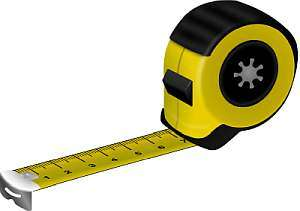# Feet and Inches to Meters Conversion Calculator

Instructions: Use this calculator to convert a lengths or heights given in feet+inches notation into meters, showing all the steps. Please type a length you would like to convert in the form below such 6'2", etc:Type a length in feet and inches (Ex: 6' 1")

## Using this Feet+Inches to Meters Conversion Calculator

There are two main dominant measuring systems: the metric system and the imperial system. The metric system uses as its basis the meter and centimeter, whereas the imperial system uses feet and inches as its basis..

Anyone who has interacted with people from other countries have experienced the fact that people may use different ways of expressing lengths and heights. For example, people from Germany will say things like "I am 1.83 meters tall", which could be a bit alien for people in the US, for example.

In places like the US and England, people are used to measure lengths and heights with feet and inches, like "6' 1", or even to define lengths using yards. Because of this variety of units, it is import to have handy metric conversion tools.## How can you convert feet+inch lengths into the metric system?

In order to conduct the conversion, you need to start with the basic knowledge that when you take a measure like 5' 11", you mean that you have 5 feet plus 11 inches. So you need to combine the conversion of these two amounts, and add up the results

## How do you do the height conversion when you have imperial units

The main objective is to first separate the amount of feet and inches, and then use a conversion formula for each them separately.

### Steps for the conversion of feet+inches to meters

1. We observe that that 39.37 inches is the same as 1 meter and 3.281 feet is the same as 1 meter. This is used as the base, initial proportion
2. Now, for a length or height that is given in feet+inches format, you need to identify the number of feet F, and the number of inches I
3. Using a direct proportion, we establish that $$\displaystyle \frac{1}{39.87} = \frac{M_1}{I}$$ and $$\displaystyle \frac{1}{3.281} = \frac{C_2}{F}$$
4. Solving the proportion for $$M_1$$ and $$M_2$$, we get the following conversion formula for meters: $$M = M_1 + M_2 = \displaystyle \frac{F}{3.281} + \frac{I}{39.87}$$

Then, all we have to do now is to plug in the corresponding values of $$F$$ and $$I$$ corresponding to the given amount you want to convert.### Examples: Converting imperial units to meters

What is 5'6 in meters?

Solution: All we have to do is to take a look at the conversion formula:

$M = \displaystyle \frac{F}{3.281} + \frac{I}{39.87}$

In this case, the given length consists of 5 feet and 6 inches, so then plugging it in the above formula we find that :

$M = \displaystyle \frac{F}{3.281} + \frac{I}{39.87} = \displaystyle \frac{5}{3.281} + \frac{6}{39.87} = 1.674 \text{ meters}$

### Another conversion example

Convert 5'9 in ms

Solution: Same as above, we need to take a look at the metric conversion formula. We have:

$M = \displaystyle \frac{F}{3.281} + \frac{I}{39.87}$

and now the given length is 5' 9, which corresponds to 5 feet and 9 inches, so we find that:

$M = \displaystyle \frac{F}{3.281} + \frac{I}{39.87} = \displaystyle \frac{5}{3.281} + \frac{9}{39.87} = 1.75 \text{ meters}$

### More unit conversions

Convert 6'2" in meters

Solution: We need to take a look at the formula:

$M = \displaystyle \frac{F}{3.281} + \frac{I}{39.87}$

In this case we have 6' 2", which is decomposed as 6 feet and 2 inches. Therefore, so we find that:

$M = \displaystyle \frac{F}{3.281} + \frac{I}{39.87} = \displaystyle \frac{6}{3.281} + \frac{2}{39.87} = 1.879 \text{ meters}$

## Other useful metric Conversion calculators

Having metric conversion calculators always come in handy. We thought that you may find useful the following calculators that are related to metric conversions, such as for example our meters to inches calculator, or the centimeters to feet calculator, or also our meters to feet, or imperial to meters calculators, depending on which ones can help you with different conversion calculations you have at hand.